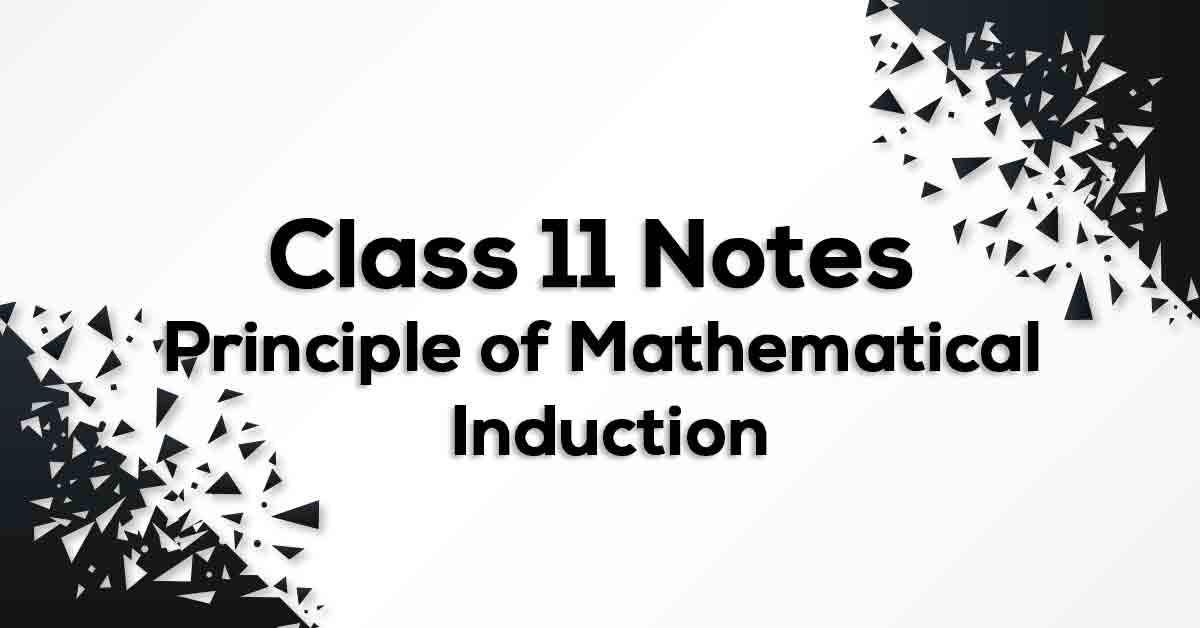Principle of Mathematical Induction Class 11 Notes | Vidyakul
×

# Principle of Mathematical Induction Class 11 Notes## Class 11 Math Chapter 4 Principle of Mathematical Induction Notes - PDF Download

Principle of Mathematical Induction Class 11 Notes are cumulated by our panel of highly experienced teachers to provide the students with effective exam preparation. The pdf not only includes the list of formulae but also offer students with the summary of the chapter, important points to remember and detailed explanation of important concepts and derivations for better understanding and retaining of the chapter. Going through these Principle of Mathematical Induction Class 11 Formulae would easily help students to grasp challenging concepts which would ultimately lead them to score maximum marks in their exams.

### Understanding Principle of Mathematical Induction.

The principle of mathematical induction is one such tool which can be used to prove a wide variety of mathematical statements. Each such statement is assumed as P(n) associated with positive integer n, for which the correctness for the case n = 1 is examined. Then assuming the truth of P(k) for some positive integer k, the truth of P (k+1) is established.

Learn how to solve such equations in Principle of Mathematical Induction Class 11 Notes pdf.